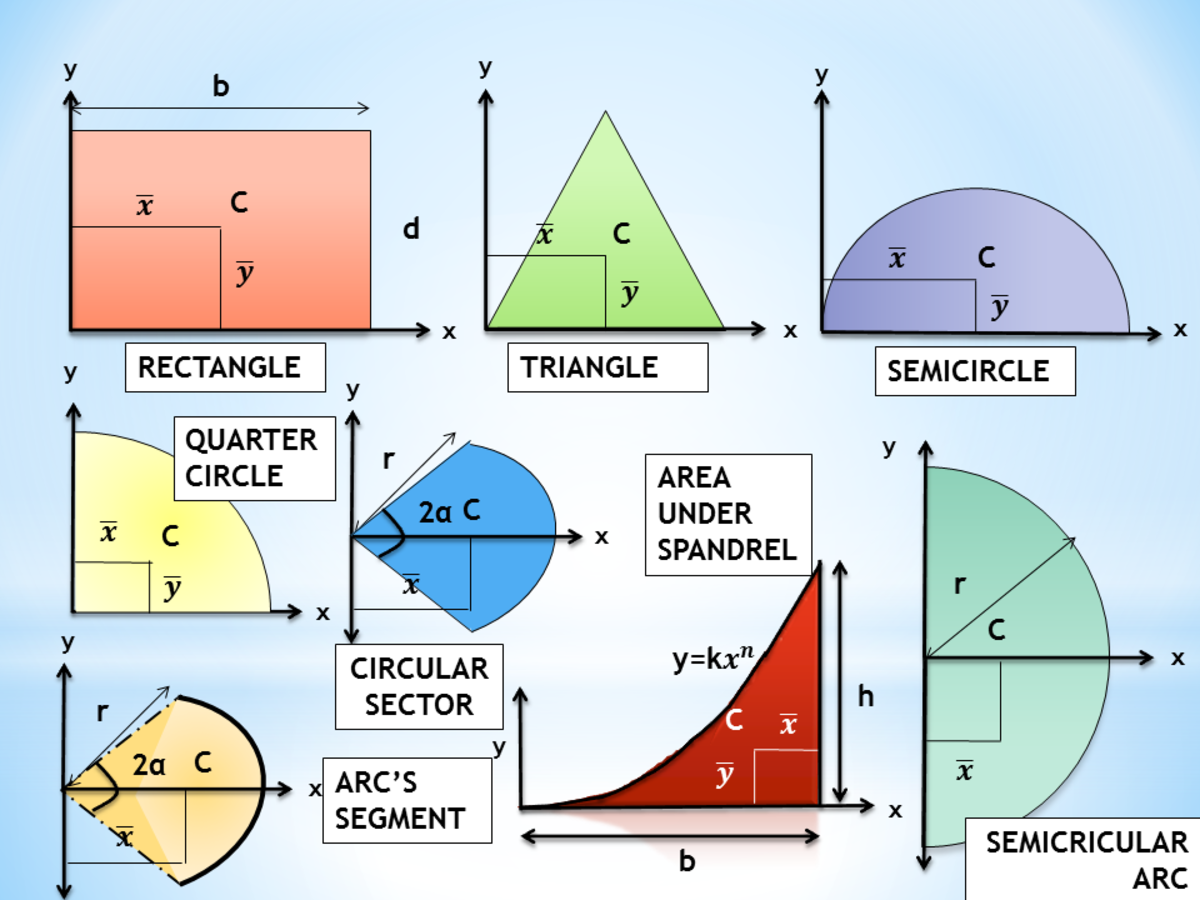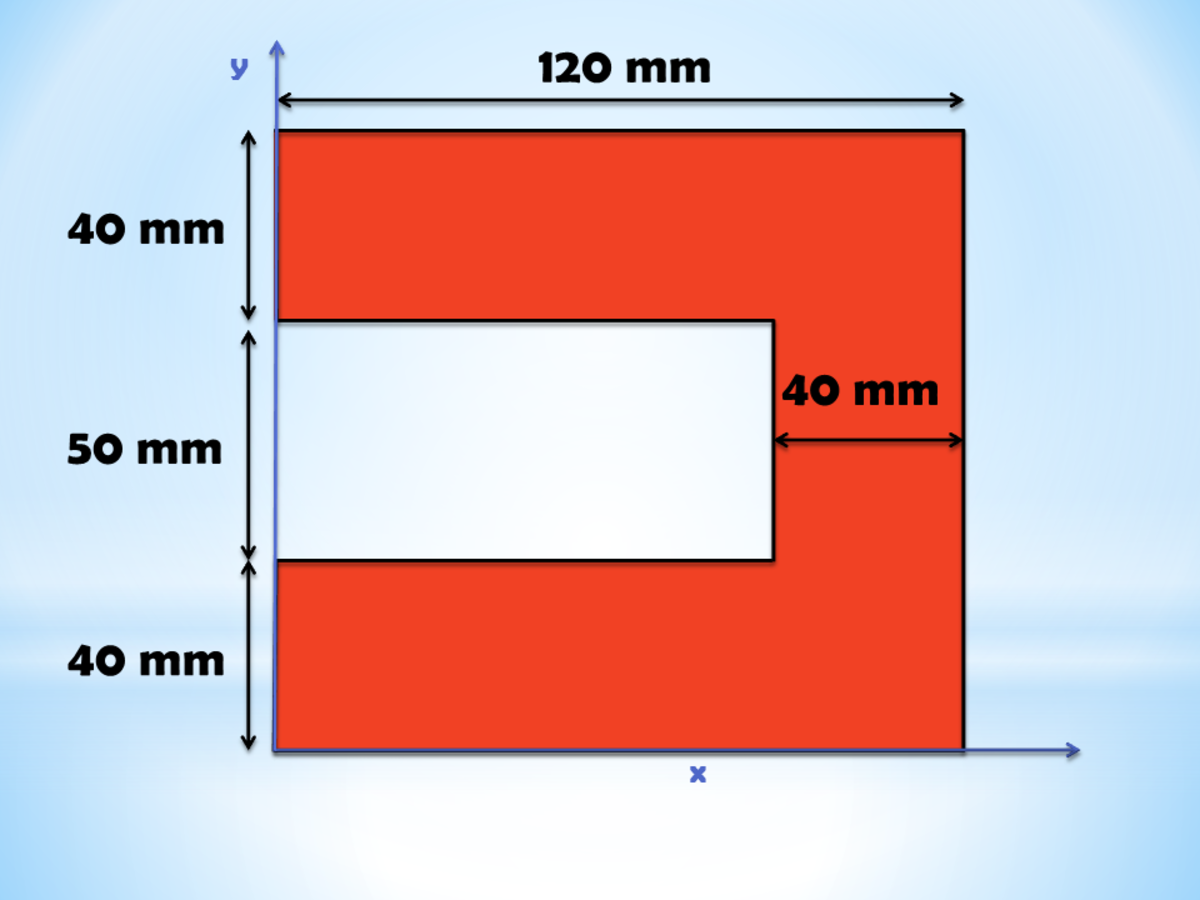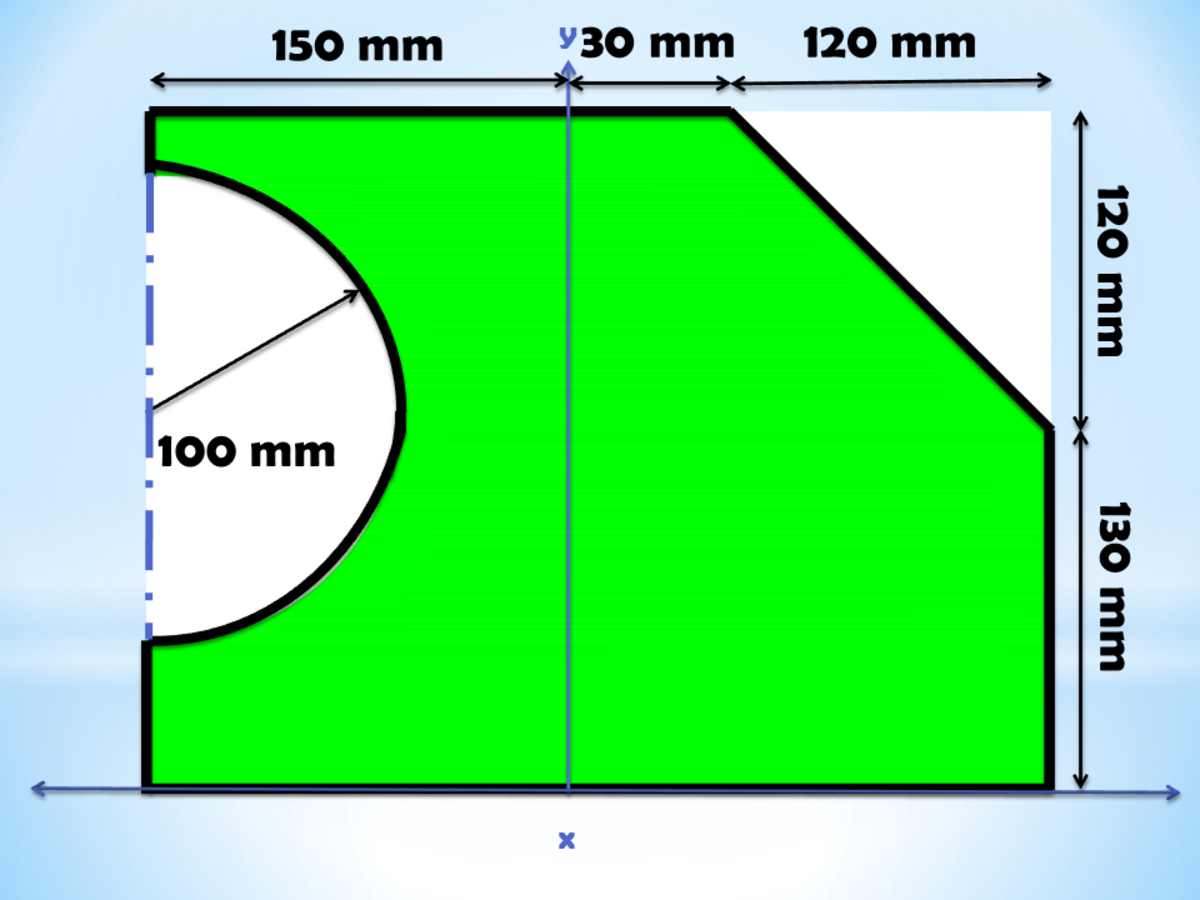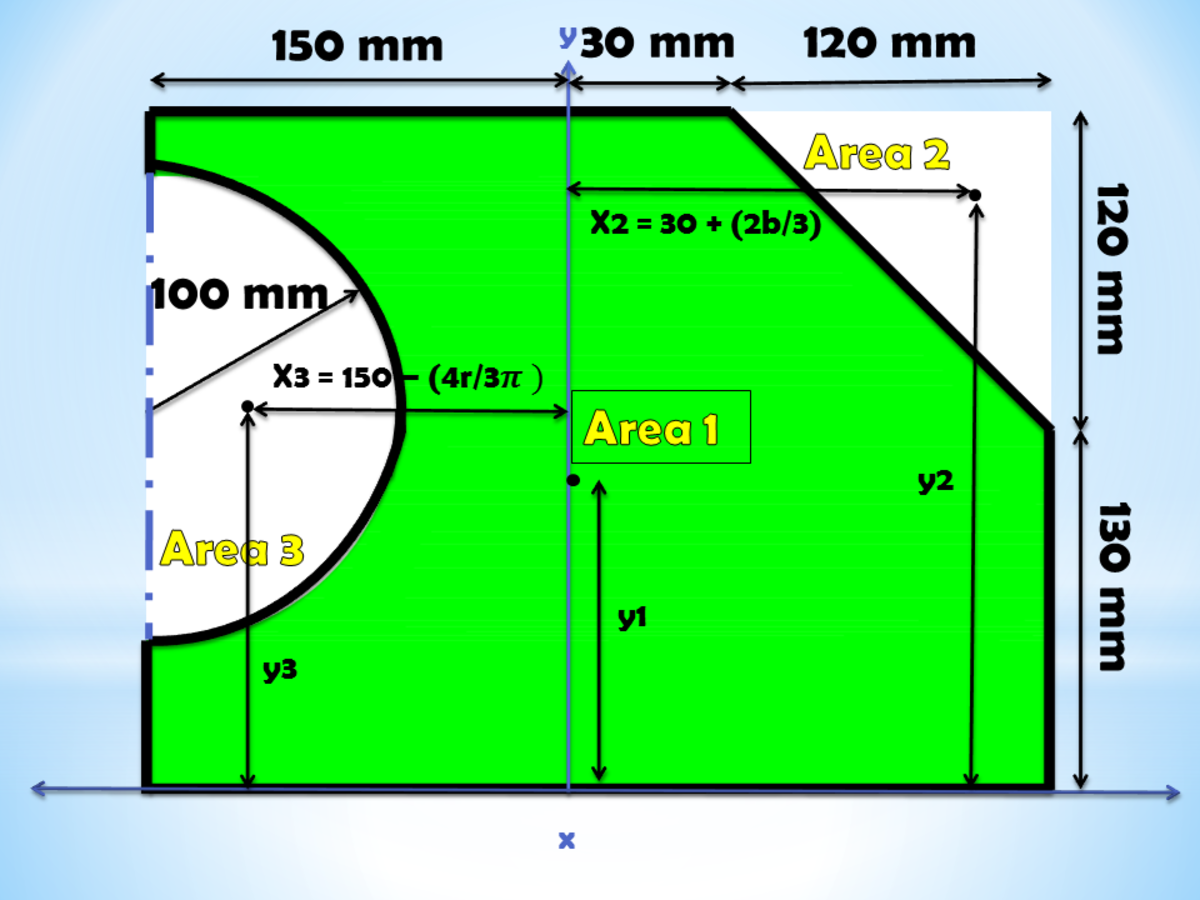# Calculating the Centroid of Compound Shapes Using the Method of Geometric Decomposition

• Author:
• Updated date:

Ray is a licensed engineer in the Philippines. He loves to write about mathematics and civil engineering.

## What Is a Centroid?

A centroid is the central point of a figure and is also called the geometric center. It is the point that matches the center of gravity of a particular shape. It is the point which corresponds to the mean position of all the points in a figure. The centroid is the term for 2-dimensional shapes. The center of mass is the term for 3-dimensional shapes. For instance, the centroid of a circle and a rectangle is in the middle. The centroid of a right triangle is 1/3 from the bottom and the right angle. But how about the centroid of compound shapes?

## What Is Geometric Decomposition?

Geometric Decomposition is one of the techniques used in obtaining the centroid of a compound shape. It is a widely used method because the computations are simple, and requires only basic mathematical principles. It is called geometric decomposition because the calculation comprises decomposing the figure into simple geometric figures. In geometric decomposition, dividing the complex figure Z is the fundamental step in calculating the centroid. Given a figure Z, obtain the centroid Ci and area Ai of each Zn part wherein all holes that extend outside the compound shape are to be treated as negative values. Lastly, compute the centroid given the formula:

Cx = ∑Cix Aix / ∑Aix

Cy = ∑Ciy Aiy / ∑Aiy

## Step-By-Step Procedure in Solving for the Centroid of Compound Shapes

Here are the series of steps in solving for the centroid of any compound shape.

1. Divide the given compound shape into various primary figures. These basic figures include rectangles, circles, semicircles, triangles and many more. In dividing the compound figure, include parts with holes. These holes are to treat as solid components yet with negative values. Make sure that you break down every part of the compound shape before proceeding to the next step.

2. Solve for the area of each divided figure. Table 1-2 below shows the formula for different basic geometric figures. After determining the area, designate a name (Area one, area two, area three, etc.) for each area. Make the area negative for designated areas that act as holes.

3. The given figure should have an x-axis and y-axis. If x and y-axes are missing, draw the axes in the most convenient means. Remember that x-axis is the horizontal axis while the y-axis is the vertical axis. You can position your axes in the middle, left, or right.

4. Get the distance of the centroid of each divided primary figure from the x-axis and y-axis. Table 1-2 below shows the centroid for different basic shapes.

## Centroid for Common Shapes

Table 1-2: Centroid for Common Shapes. X-bar is the distance of the centroid from the y-axis. Y-bar is the distance of the centroid from the x-axis.

ShapeAreaX-barY-bar

Rectangle

bh

b / 2

d / 2

Triangle

(bh) / 2

-

h / 3

Right triangle

(bh) / 2

h / 3

h / 3

Semicircle

(pi (r^2)) / 2

0

(4r) / (3(pi))

Quarter circle

(pi (r^2)) / 4

(4r) / (3(pi))

(4r) / (3(pi))

Circular sector

(r^2) (alpha)

(2rsin(alpha)) / 3(alpha)

0

Segment of arc

2r(alpha)

(rsin(alpha)) / alpha

0

Semicircular arc

(pi) (r)

(2r) / pi

0

Area under spandrel

(bh) / (n + 1)

b / (n+2)

(hn + h) / (4n + 2)Centroids of Simple Geometric ShapesJohn Ray Cuevas

5. Creating a table always makes computations easier. Plot a table like the one below.

Table 1-1: Table Format. X is the distance of the centroid from the y-axis. Y is the distance of the centroid from the x-axis.

Area NameArea (A)xyAxAy

Area 1

-

-

-

Ax1

Ay1

Area 2

-

-

-

Ax2

Ay2

Area n

-

-

-

Axn

Ayn

Total

(Total Area)

-

-

(Summation of Ax)

(Summation of Ay)

6. Multiply the area 'A' of each basic shape by the distance of the centroids 'x' from the y-axis. Then get the summation ΣAx. Refer to the table format above.

Scroll to Continue

## Read More From Owlcation

7. Multiply the area 'A' of each basic shape by the distance of the centroids 'y' from the x-axis. Then get the summation ΣAy. Refer to the table format above.

8. Solve for the total area ΣA of the whole figure.

9. Solve for the centroid Cxof the whole figure by dividing the summation ΣAx by the total area of the figure ΣA. The resulting answer is the distance of the entire figure's centroid from the y-axis.

10. Solve for the centroid Cy of the whole figure by dividing the summation ΣAy by the total area of the figure ΣA. The resulting answer is the distance of the entire figure's centroid from the x-axis.

Here are some examples of obtaining a centroid.Centroid for Complex Figures: C-shapesJohn Ray Cuevas

## Problem 1: Centroid of C-Shapes

Solution 1

a. Divide the compound shape into basic shapes. In this case, the C-shape has three rectangles. Name the three divisions as Area 1, Area 2, and Area 3.

b. Solve for the area of each division. The rectangles have dimensions 120 x 40, 40 x 50, 120 x 40 for Area 1, Area 2, and Area 3 respectively.

c. X and Y distances of each area. X distances are the distances of each area's centroid from the y-axis, and Y distances are the distances of each area's centroid from the x-axis.

d. Solve for the Ax values. Multiply the area of each region by the distances from the y-axis.

e. Solve for the Ay values. Multiply the area of each region by the distances from the x-axis.

All units are in terms of millimeters.

Area NameArea (A)xyAxAy

Area 1

4800

60

20

288000

96000

Area 2

2000

100

65

200000

130000

Area 3

4800

60

110

288000

528000

Total

11600

776000

754000

f. Finally, solve for the centroid (Cx, Cy) by dividing ∑Ax by ∑A, and ∑Ay by ∑A.

The centroid of the complex figure is 66.90 millimeters from the y-axis and 65.00 millimeters from the x-axis.Centroid for Complex Figures: Irregular figuresJohn Ray Cuevas

## Problem 2: Centroid of Irregular Figures

Solution 2

a. Divide the compound shape into basic shapes. In this case, the irregular shape has a semicircle, rectangle, and right triangle. Name the three divisions as Area 1, Area 2, and Area 3.

b. Solve for the area of each division. The dimensions are 250 x 300 for the rectangle, 120 x 120 for the right triangle, and a radius of 100 for the semicircle. Make sure to negate the values for the right triangle and semicircle because they are holes.

c. X and Y distances of each area. X distances are the distances of each area's centroid from the y-axis, and y distances are the distances of each area's centroid from the x-axis. Consider the orientation of x and y-axes. For Quadrant I, x and y are positive. For Quadrant II, x is negative while y is positive.Solution for Irregular ShapeJohn Ray Cuevas

d. Solve for the Ax values. Multiply the area of each region by the distances from the y-axis.

e. Solve for the Ay values. Multiply the area of each region by the distances from the x-axis.

All units are in terms of millimeters.

Area NameArea (A)xyAxAy

Area 1

75000

0

125

0

9375000

Area 2

- 7200

110

210

-792000

-1512000

Area 3

- 5000pi

- 107.56

135

1689548.529

-2120575.041

Total

52092.04

897548.529

5742424.959

f. Finally, solve for the centroid (Cx, Cy) by dividing ∑Ax by ∑A, and ∑Ay by ∑A.

The centroid of the complex figure is 17.23 millimeters from the y-axis and 110.24 millimeters from the x-axis.

## Moment of Inertia of Irregular or Compound Shapes

This content is accurate and true to the best of the author’s knowledge and is not meant to substitute for formal and individualized advice from a qualified professional.

## Questions & Answers

Question: Is there any alternative method for solving for the centroid except this geometric decomposition?

Answer: Yes, there is a technique using your scientific calculator in solving for the centroid.

Question: in area two of triangle in problem 2...how 210mm of y bar has obtained?

Answer: It is the y-distance of the centroid of the right triangle from the x-axis.

y = 130 mm + (2/3) (120) mm

y = 210 mm

Question: Regarding Q1, there are graphical methods that can be used in many simple cases. Have you seen the game app, Pythagorean?

Answer: It looks interesting. It says that Pythagorea is a collection of geometric puzzles of different kind that can be solved without complex constructions or calculations. All objects are drawn on a grid whose cells are squares. A lot of levels can be solved using just your geometric intuition or by finding natural laws, regularity, and symmetry. This could really be helpful.

Question: How did the y-bar for area 3 become 135 millimeters?

Answer: I am very sorry for the confusion with the computation of the y-bar. There must be some dimensions lacking in the figure. But as long as you understand the process of solving problems about centroid, then there's nothing to worry about.

Question: How do you calculate w-beam centroid?

Answer: W-beams are H/I beams. You can start solving the centroid of a W-beam by dividing the whole cross-sectional area of the beam into three rectangular areas - top, middle, and bottom. Then, you can start following the steps discussed above.

Question: In problem 2, why is the quadrant positioned at the middle and the quadrant in problem 1 is not?

Answer: Most of the time, the position of the quadrants is given in the given figure. But in case that you are asked to do it yourself, then you should place the axis to a position where you can solve the problem in the most easy way. In problem number two's case, placing the y-axis at the middle will yield to an easier and short solution.

JR Cuevas on August 23, 2020:

Hi, Luke and Dexter! Unfortunately, there is a lacking dimension on the figure. But you can follow this:

Given radius of 100 mm, assume 35 mm from the bottom up to the first point of the semi-circle.

100 mm + 35 mm = 135 mm from the bottom of the figure up to the centroid of the semi-circle.

I am very sorry for the inconvenience.

luke on August 23, 2020:

how did you get you y value for area 3 ?

Dexter on August 23, 2020:

How did you get the y-bar for area 3

JackieZhang on June 30, 2020:

Thank you! Very very easy to understand!

Trying boy on June 11, 2020:

Nice. Easy to understand. Good explaination!

Ray (author) from Philippines on March 08, 2020:

Please, check on the "Questions and Answers" portion of the article to look for similar questions. Thanks!

Ray (author) from Philippines on January 23, 2020:

Hi, Good day Srikar! H/3 is the distance of the centroid of the triangle from the base of the triangle while 2H/3 is the distance of the centroid of the triangle from the vertex or tip of the triangle.

Srikar on January 23, 2020:

When to use b/3 and 2b/3 for x of triangle

Ray (author) from Philippines on December 17, 2019:

Hi, Mousa. I am very sorry for the confusion with the computation of the y-bar. There must be some dimensions lacking in the figure. But as long as you understand the process of solving problems about centroid, then there's nothing to worry about.

Mousa Alzahrani on December 17, 2019:

in area three in problem 2... how 135 mm of y bar has obtained ?

K on November 01, 2019:

Sooper

Faiz on January 11, 2019:

Why centroid is out of fig in c shpae??